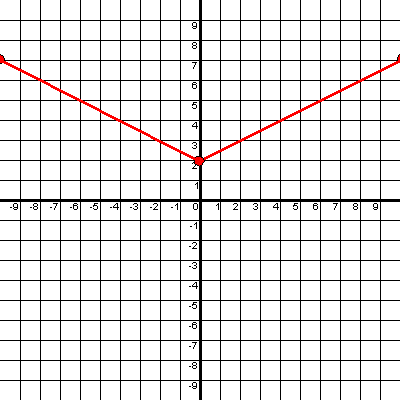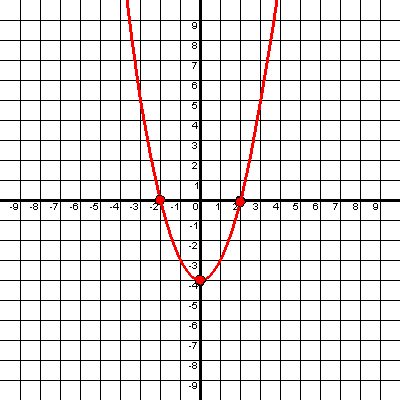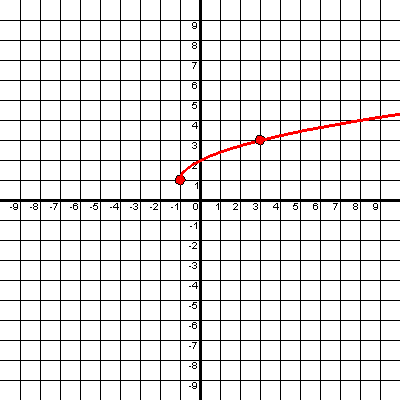Graphing Examples

### Graphing Examples:

Here are some examples of the graphs you can create with my graphing tools. They are: a number line grapher, a grapher for linear equations and inequalities, and a tool for creating graphs of absolute value, quadratic (parabolas), and square root functions.

With the number line grapher you can create a graph to represent the solution set of an inequality in one variable like this: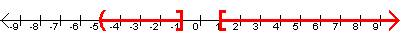The grapher for linear equations and inequalities allows you to create a graph of a line in two variables: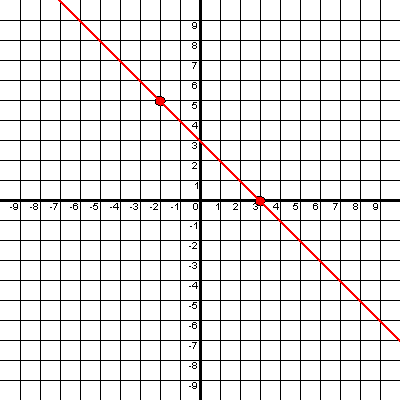or a graph of a linear inequality in two variables: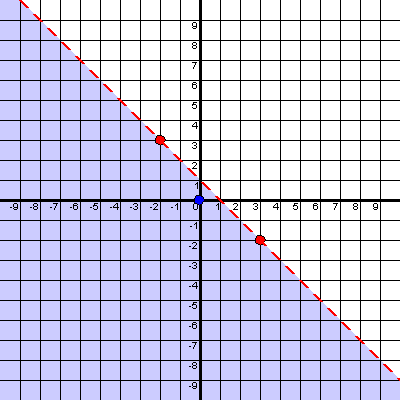or a graph of a system of linear inequalities in two variables: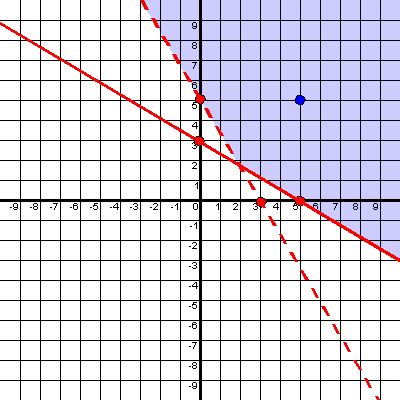With the tool for creating graphs of absolute value, quadratic (parabolas), and square root functions you can create graphs like these:

Absolute value function: